Start typing, then use the up and down arrows to select an option from the list.# Half-Life Example 2

Jules Bruno
121views
here, we're told that the rate constant for the following reaction was found to be 2.3 times 10 to negative three seconds inverse at 35 degrees Celsius. Here, if the initial concentration of nitrogen dioxide is 1.4 times 10 to the negative one, what is the half life of this reaction? Or on this reaction? Remember since our rate constant K. Has units of just time in verse. This is a giveaway that it is a first order rate law process. And since its first order, that means half life equals Ln two over K. So, Alan two divided by a rate constant K. Which is 2.3 times 10 to the -3 seconds in verse When we punch that in, that gives us 301 seconds. This would be our final answer. Now, notice what else did I give us? I gave us the initial concentration of our reactant. But remember that's nowhere to be seen within our formula for half life for a first order rate law process. So, that's just extra information given to us at times were given a lot of info and a question that doesn't necessarily mean we have to use all of it. This is one of those times. The only part that's important here is our rate constant K. Which we plug in and that helps us to find our half life at the very end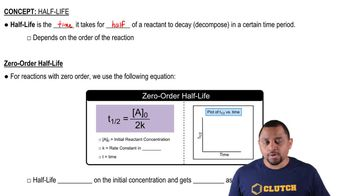02:17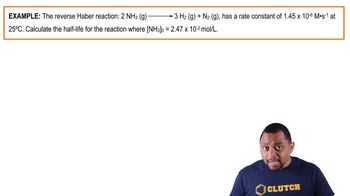01:19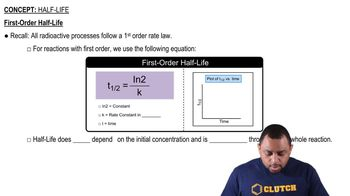01:49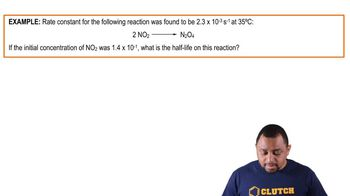01:18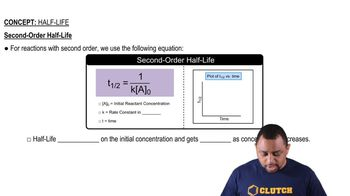01:44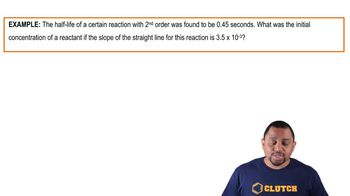01:52